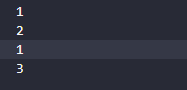# Lua-基础（三）函数

### 函数语法

``````function func_name (arguments-list)
statements-list
end
``````

Ps：Lua 使用的函数可以是 Lua 编写也可以是其他语言编写，对于 Lua 程序员来说用什

``````function Show(a)
print(a)
end
Show(100)
``````

#### 返回多个值

（如果不存在匹配串返回 nil）。

``````s, e = string.find("hello Lua users", "Lua")
print(s, e)  --> 7 9
``````

``````function MaxM(arr)
local maxIndex=1
local max=arr[maxIndex]
for key, value in pairs(arr) do
if value>max then
maxIndex=key
max=value
end
end
return max,maxIndex
end
local max,maxIndex=MaxM({1.5,6,7,9})
print('最大值和索引为:'..max..'  '..maxIndex)
``````

#### 返回函数

`return fac()`这样的形式就是返回函数，其意义是执行fac函数并返回fac的返回值，若没有返回nil。

#### 可变参数

##### unpack

`unpack`，接受一个数组作为输入参数，返回数组的所有元素。

``````f = string.find
a = {"hello", "ll"}
print(f(unpack(a))) --> 3 4
``````
##### ...可变参数

``````function Sum(a,b,c,...)
local arg={...} --将可变参数存储到arg表中
for key, value in pairs(arg) do
print(value) -- 9 7 4 10
end
end

Sum(5,4,8,9,7,4,10)
``````

#### 使用命名参数

``````function Show(arg)
print(arg.name..'  '..arg.title)
end

Show{name='小屋',title='学习lua'}
``````

### 深入理解函数

Lua 中的函数是带有词法定界（lexical scoping）的第一类值（first-class values）。

#### 匿名函数

`table.sort()`函数中使用匿名函数：

``````arr={
{name='A',age=16},
{name='B',age=20},
{name='C',age=9},
}
table.sort(arr,function (a,b)
return a.age>b.age
end)

for key, value in pairs(arr) do
for k, v in pairs(value) do
print(k,v)
end
end
``````

#### 闭包

``````function NewCount()
local i=0
return function ()
i=i+1
return i
end
end
``````

``````local c1=NewCount()
local c2=NewCount()
print(c1())
print(c1())
print(c2())
print(c1())
``````Ps：我们把这个i称为外部的局部变量或者 upvalue

#### 使用闭包重写函数

``````local oldSin=math.sin
math.sin=function (x)
return oldSin(x*math.pi/180)
end
``````

#### 局部函数

Lua中函数既可以作为全局变量也可以作为局部变量，函数作为table的域。

``````Lib={}
Lib.foo=function (x,y)
return x+y
end
Lib.goo=function (x,y)
return x-y
end

Lib={
foo=function (x,y)
return x+y
end,
goo=function (x,y)
return x-y
end
}

Lib={}
function Lib.foo(x,y)
return x+y
end
function Lib.goo(x,y)
return x-y
end

``````

Ps：在使用递归函数时，要注意要先声明函数在调用。

#### 尾调用

Ps:Lua中尾调用不消耗栈空间，由于尾调用不需要使用栈空间，那么尾调用递归的层次可以无限制的